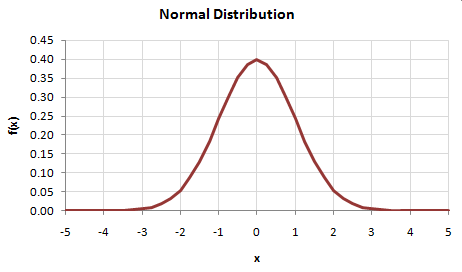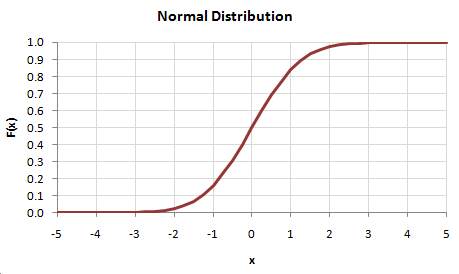# HOMER Pro 3.9A probability transformation is a statistical procedure by which one modifies a set of numbers to conform to a desired probability distribution function.

To perform a probability transformation, HOMER first calculates the cumulative distribution function of the original set of data -- we will refer to this as the 'original CDF'. Then for each original data point, it performs the following steps:

1.It refers to the original CDF to calculate the percentile value corresponding to that original data point

2.It refers to the desired CDF to calculate the transformed value corresponding to that same percentile value

Let's look at an example to illustrate this process. Imagine that we have a set of data that conform to a normal distribution, and we want to transform it so that it conforms to a Weibull distribution. (HOMER does exactly this when synthesizing wind speed data.)

If our normally-distributed data had a mean of zero and a standard deviation of 1, its probability distribution function would look like so:And its cumulative distribution function -- the original CDF -- would look like so:Imagine that we wish to transform this data to fit a Weibull distribution with a mean value of 6 and a Weibull k value of 2. Our desired probability distribution function would therefore look like so:And our desired cumulative distribution function -- the desired CDF -- would look like so:To transform each value in the original data set, we would refer to the original CDF to find its corresponding y-value, then we would take that same y-value to the desired CDF and find its corresponding x-value.

An original value of zero, for example, corresponds to a CDF value of 0.5 on the original CDF. Looking at the desired CDF, we find that the value corresponding to a CDF value of 0.5 is approximately 5. That means that any zero value in the original data set gets transformed into a value of 5 in the transformed data set. Similarly, an original value of -1 would be transformed to value of approximately 2.5, and an original value of 1.5 would be transformed to a value of approximately 10.

This example looks at transforming data from a normal distribution to a Weibull distribution, but with this same probability transformation approach, we could transform from any distribution to any other distribution.